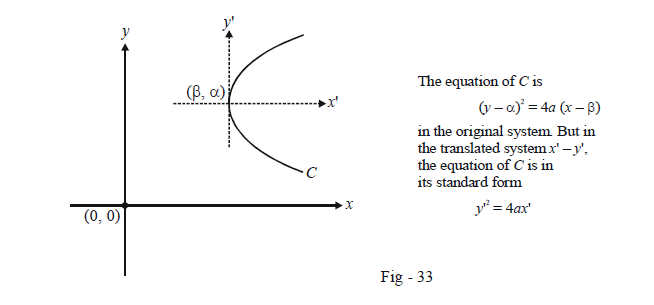# Parabola Equations For Shifted Axes

## CONCLUDING NOTE

Sometimes, the equation of the parabola might not be in the standard form $${y^2} = 4ax{\rm{ }}\;or\;{\rm{ }}{x^2} = 4ay.$$ or However, by a transformation (translation and / or rotation) of the co-ordinate axes, the equation given can always be reduced to the standard form.

For example, suppose that the equation of a (translated) parabola is

${(y - \alpha )^2} = 4a(x - \beta )$

The vertex of this parabola is at $$(\beta ,\alpha )$$ instead of (0, 0). Thus, we cannot use all the formulae that we’ve derived directly on this form. However, by a translation of the axis, so that the new origin coincides with $$(\beta ,\alpha ),$$ i.e., by the transformation

$y \to y' + \alpha ,\,\,\,\,x \to x' + \beta$

the equation of the parabola referred to the new axes will become

${y'^2} = 4ax'$

which is indeed in the standard form. We can now use this form to evaluate whatever we were required to, say, some tangent or some normal in the $$x' - y'$$ axes. Once we have the required equation, we can convert it to the x – y system using the reverse transformations

$y' \to y - \alpha ,\,\,\,\,\,x' \to x - \beta$As a very simple example, suppose that we have to find the focus and directrix of the parabola

${y^2} - 4x - 6y + 25 = 0$

which can be rearranged as

${(y - 3)^2} = 4(x - 4) \qquad \dots \left( 1 \right)$

Thus, a = 1. However, we cannot directly use (a, 0) as the focus which should be obvious.

Referred to the co-ordinate system $$x' - y'{\rm{ }}\;where\;{\rm{ }}x' = x - 4{\rm{ }}\;and\;{\rm{ }}y' = y - 3,$$ where and the equation (1) is

${y'^2} = 4x'$

It is in this system that the focus will be at (a, 0), i.e. at (1, 0). Thus, $${x'_f} = 1{\rm{ }}\;and\;{\rm{ }}{y'_f} = 0$$ and . In the original system, the focus can be evaluated by the reverse transformation

\begin{align}&\qquad \quad \; \; x \;= 4 + x' \qquad {\rm{and }}\qquad y \;\,= 3 + y'\\&\Rightarrow \qquad {x_f} = 4 + {{x'}_f}\quad\;\,{\rm{ and}} \qquad {\rm{ }}{y_f} = 3 + {{y'}_f}\\&\qquad \qquad \;\;{\rm{ }} = {\rm{ }}5\;\;\;\qquad \qquad\qquad \quad{\rm{ }} \;\;\,= {\rm{ }}3\end{align}

Thus, the focus in the original system is at (5, 3). Of course, this example is trivial and the answer could have been evaluated simply be inspection. However, it was described just to highlight the underlying approach you could follow in such cases.

Let us now consider a non-trivial example using this approach. Suppose that we have to find all the points on the axis of the parabola $$3{y^2} + 4y - 6x + 8 = 0$$ from where three distinct normals can be drawn to the parabola. Assume such points to be (h, k). The equation of the parabola can be rearranged as

${\left( {y + \frac{2}{3}} \right)^2} = 2\left( {x - \frac{{10}}{9}} \right)$

which can be written in the standard form using the transformations

$X = x - \frac{{10}}{9},\,\,\,\,\,Y = y + \frac{2}{3}$

as

${Y^2} = 2X$

Thus,

$a = \frac{1}{2}$

We will now work in the X-Y system. Any point on the axis of the parabola can be taken as (p, 0) (in this new system). The equation of an arbitrary normal to the parabola $${Y^2} = 2X$$ is

$Y = mX - m - \frac{{{m^3}}}{2}$

If this passes through (p, 0), we have

\begin{align}& \qquad \;\;mp - m - \frac{{{m^3}}}{2} = 0\\\\&\Rightarrow \quad m\left( {\frac{{{m^2}}}{2} + 1 - p} \right) = 0\\\\&\Rightarrow \quad m = 0,\,\,\,\,\, \pm \sqrt {2(p - 1)}\end{align}

Thus, we have three distinct normals if

\begin{align}& \qquad \;\; \quad p - 1 > 0\\&\Rightarrow \qquad p > 1\end{align}

Therefore, three distinct normals can be drawn to the parabola from $$(p,0)$$ if p > 1.

However, note that this result is in the X – Y system and not the x – y system. To obtain the result in the original system, we must use the reverse transformations

$X = x - \frac{{10}}{9},\,\,\,\,\,Y = y + \frac{2}{3}$

Thus, if as assumed earlier, the required points in the original system are (h, k) (which are (p,0) in the new system) we have

$p = h - \frac{{10}}{9}\,\,\,\,\,0 = k + \frac{2}{3}$

Since p > 1, we have \begin{align}h > \frac{{19}}{9}. {\rm{Also,}} \; k = - \frac{2}{3}\end{align}.

Thus, from all points on the axis, \begin{align}\left( {h, - \frac{2}{3}} \right), {\rm{where }}\;\;h > \frac{{19}}{9}\end{align} , three distinct normals can be drawn to the parabola.

If you did not understand this discussion, you are urged to re-read it until you do.

## TRY YOURSELF – IV

 Q. 1 Find the locus of the mid-points of the chords of the parabola $${y^2} = 4ax$$ which subtend a right angle at the focus (using a homogenizing approach.) Q. 2 Prove that the locus of the mid-point of a system of parallel chords of a parabola is a line parallel to the axis of the parabola. Q. 3 What is the equation of the chord of contact of the tangents drawn from $$P( - 1,\,\,2)$$ to $${y^2} = 4x\,\,?$$ Q. 4 Prove the pole of an external point P is the chord of contact of the tangents drawn from P to the parabola. Q. 5 From the pole P of a chord AB of a parabola, a line is drawn parallel to the axis of the parabola. This line intersects the parabola in Q and AB in R. Prove that PQ = QR.